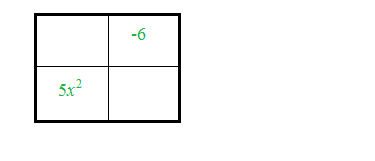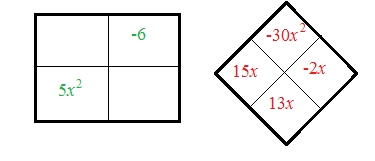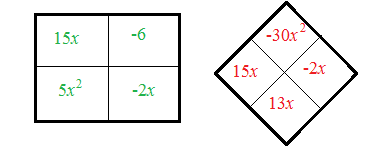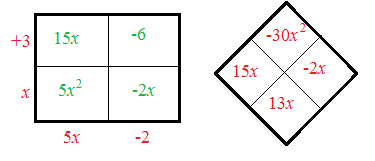### Home > AC > Chapter 9 > Lesson 9.3.1 > Problem9-65

9-65.

1. $5x^{2} + 13x − 6$

Create a generic rectangle.

Use the diamond pattern from previous chapters.

Find the missing pieces of the diamond.

Fill in the generic rectangle.

Find the factors.

5x² + 13x − 6 = (5x − 2)(x + 3)2. $6t^{2} − 26t + 8$

Start by factoring out the GCF.

3. $6x^{2} − 24$

Factor out a 6.
You will be left with a difference of squares.